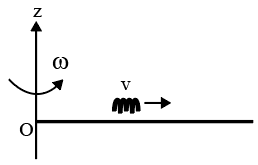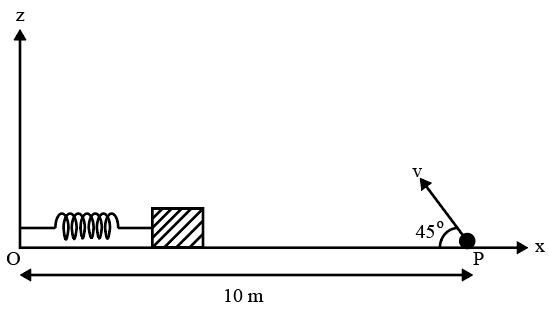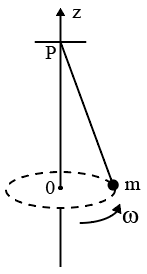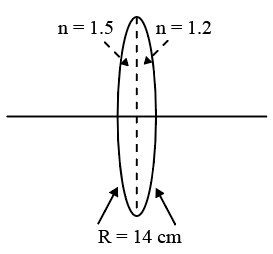Instructions

For the following questions answer them individually

Question 1

# Athin uniform rod, pivoted at O,is rotating in the horizontal plane with constant angular speed $$\omega$$, as shownin the figure. At time t = 0, a small insect starts from O and moves with constant speedv with respect to the rod towardsthe other end. It reaches the end of the rod at t = T and stops. The angular speed of the system remains $$\omega$$ throughout. The magnitude of the torque $$(\mid \overrightarrow{\tau} \mid)$$ on the system about O, as a function of time is best represented by which plot ?Question 2

# Three very large plates of same area are kept parallel and close to each other. They are considered as ideal black surfaces and have very high thermal conductivity. Thefirst andthird plates are maintained at temperatures 27 and 37 respectively. The temperature of the middle (i.e. second) plate under steady state condition isQuestion 3

# Consider a thin spherical shell of radius R with its centre at the origin, carrying uniform positive surface charge density. The variation of the magnitude of the electric field $$\mid \overrightarrow{E}(r) \mid$$ and the electric potential V(r) with the distance r from the centre, is best represented by which graph?Question 4

# In the determination of Young’s modulus $$\left(Y = \frac{4MLg}{\pi l d^2}\right)$$ by using Searle’s method,a wire of length L=2 mand diameter d=0.5 mmis used. For a load M= 2.5 kg, an extension l = 0.25 mm in the length of the wire is observed. Quantities d and/ are measured using a screw gauge and a micrometer, respectively. They have the same pitch of 0.5 mm. The number ofdivisions on their circular scale is 100. The contributions to the maximum probable error of the Y measurementQuestion 5

# A small block is connected to one end of a massless spring of un-stretched length 4.9 m. The other end of the spring (See thefigure)is fixed. The system lies on a horizontal frictionless surface. The blockis stretched by 0.2 m and released from rest at t= 0. It then executes simple harmonic motion with angular frequency $$\omega = \frac{\pi}{3}$$ rad/s. Simultaneously at t = 0, a small pebble is projected with speed v from point P at an angle of $$45^\circ$$ as shownin thefigure. Point P is at a horizontal distance of 10 m from O. If the pebble hits the block at + = 1s, the value of v is (take g = 10 $$m/s^2$$)Question 6

# Young's double slit experimentis carried out by using green, red and bluelight, one color at a time. The fringe widths recorded are $$\beta_G, \beta_R, \beta_B$$, respectively. Then,Question 7

# A small mass m is attached to a massless string whose other end is fixed at P as shown in the figure. The mass is undergoing circular motion in the x-y plane with centre at O and constant angular speed $$\omega$$ If the angular momentum of the system, calculated about O and P are denoted by $$\overrightarrow{L_O}$$ and $$\overrightarrow{L_P}$$ respectively, thenQuestion 8

# A mixture of 2 moles of helium gas (atomic mass = 4 amu) and 1 mole of argon gas (atomic mass = 40 amu) is kept at 300 K in a container. The ratio of the rms speeds$$\left(\frac{v_{rms}(helium)}{v_{rms}(argon)}\right)$$Question 9

# Two large vertical and parallel metal plates having a separation of 1 cm are connected to a DC voltage source of potential difference X . A protonis released at rest midway between the two plates. It is found to move at $$45^\circ$$ to the vertical JUSTafter release. Then X is nearlyQuestion 10

# A bi-convex lens is formed with two thin plano-convex lenses as shown in the figure. Refractive index n of the first lens is 1.5 and that of the second lens is 1.2. Both the curved surfaces are of the same radius of curvature R = 14 cm. For this bi-convex lens, for an object distance of 40 cm, the image distance will beOR